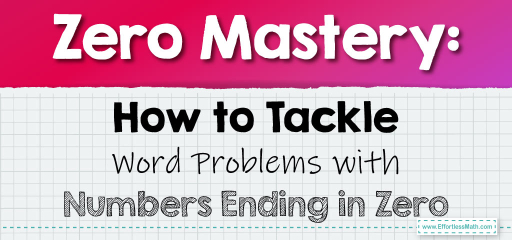# Zero Mastery: How to Tackle Word Problems with Numbers Ending in Zero

In real-world scenarios, we often encounter situations where we need to divide large numbers, especially those ending in zeros. These numbers, being multiples of 10, have unique properties that can simplify our calculations. Let's explore some word problems that involve dividing numbers ending in zero.## Dividing Numbers Ending in Zero in Word Problems

### Example 1:

A school printed $$1,000$$ brochures to distribute equally among $$20$$ classrooms. How many brochures will each classroom receive?

Solution Process:

Divide $$1,000$$ by $$20$$. Given both numbers end in zero, we can simplify this to $$100 \div 2$$.

Each classroom will receive $$50$$ brochures.

The Absolute Best Book for 5th Grade Students

### Example 2:

A farmer has $$6,000$$ kg of grains and wants to pack them in bags of $$30$$ kg each. How many bags will he need?

Solution Process:

Divide $$6,000$$ by $$30$$. Simplifying, we get $$600 \div 3$$.

The farmer will need $$200$$ bags.

Dividing numbers that end in zeros can initially seem daunting due to their size. However, by recognizing the underlying patterns and simplifying the division, these problems become much more manageable. Always remember to break down the problem, simplify where possible, and use the properties of numbers ending in zeros to your advantage. With practice, you’ll become adept at solving these real-world challenges efficiently!

### Practice Questions:

1. A library received $$5,000$$ books and wants to distribute them equally among $$25$$ shelves. How many books will each shelf have?

2. A factory produces $$8,000$$ toys and wants to pack them in boxes of $$40$$ each. How many boxes will they prepare?

3. A marathon event has $$7,000$$ water bottles to be distributed equally among $$50$$ hydration stations. How many bottles will each station get?

4. A school has $$9,000$$ pencils and wants to distribute them equally in packs of $$90$$. How many packs will they make?

5. A bakery baked $$10,000$$ cookies and plans to sell them in jars of $$100$$. How many jars will they need?

A Perfect Book for Grade 5 Math Word Problems!

1. $$200$$ books per shelf

2. $$200$$ boxes

3. $$140$$ bottles per station

4. $$100$$ packs

5. $$100$$ jars

The Best Math Books for Elementary Students

### What people say about "Zero Mastery: How to Tackle Word Problems with Numbers Ending in Zero - Effortless Math: We Help Students Learn to LOVE Mathematics"?

No one replied yet.

X
51% OFF

Limited time only!

Save Over 51%

SAVE $15 It was$29.99 now it is \$14.99